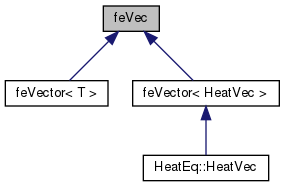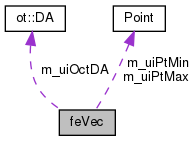Dendro  5.01 Dendro in Greek language means tree. The Dendro library is a large scale (262K cores on ORNL's Titan) distributed memory adaptive octree framework. The main goal of Dendro is to perform large scale multiphysics simulations efficeiently in mordern supercomputers. Dendro consists of efficient parallel data structures and algorithms to perform variational ( finite element) methods and finite difference mthods on 2:1 balanced arbitary adaptive octrees which enables the users to perform simulations raning from black holes (binary black hole mergers) to blood flow in human body, where applications ranging from relativity, astrophysics to biomedical engineering.
feVec Class Referenceabstract
Inheritance diagram for feVec:[legend]
Collaboration diagram for feVec:[legend]

## Public Member Functions

feVec (ot::DA *da)
: feVec constructor More...

~feVec ()
deconstructor

virtual void computeVec (const VECType *in, VECType *out, double scale=1.0)=0
Evaluates the RHS of the PDE at specific points (for example evaluation at the quadrature points) More...

void setProblemDimensions (const Point &pt_min, const Point &pt_max)
set the problem dimension

virtual void setPlaceholder (const double *v)

## Protected Attributes

ot::DAm_uiOctDA
: pointer to OCT DA

ot::DAType m_uiDaType
: type of the DA

Point m_uiPtMin
problem domain min point

Point m_uiPtMax
problem domain max point

## ◆ feVec()

 feVec::feVec ( ot::DA * da )
inline

: feVec constructor

[in] daType: type of the DA

## ◆ computeVec()

 virtual void feVec::computeVec ( const VECType * in, VECType * out, double scale = `1.0` )
pure virtual

Evaluates the RHS of the PDE at specific points (for example evaluation at the quadrature points)

Parameters
 [out] out : function evaluated at specific points. Evaluates the right hand side of the weak formulations. Typically the mass matrix multiplied with the load function. [in] in Input vector (f) [out] out : Output vector (Mf)

Implemented in feVector< T >, and feVector< HeatVec >.

The documentation for this class was generated from the following file: